# 比Linus更厲害的程序員，這家伙一定是穿越回來的

Linus Torvalds是個非常厲害的程序員，因為他有兩個名揚天下的作品：Linux和Git。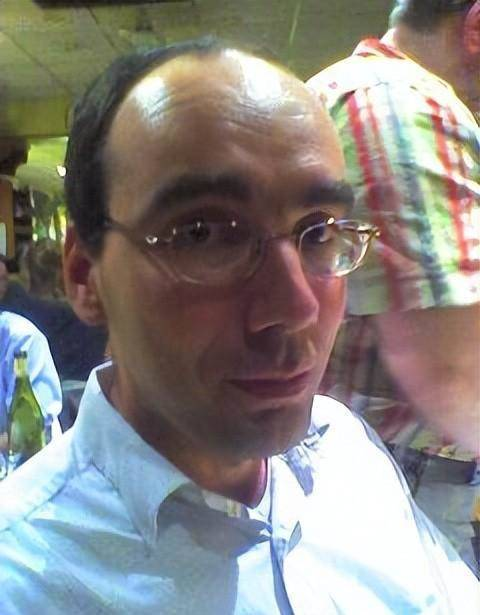## 壓縮軟件

Fabrice Bellard出生于法國東南部的一個小城市格勒諾布爾，17歲上高中的時候發現自己的電腦硬盤又小又貴，他就想著如何節省硬盤空間，于是用匯編語言開發了一個壓縮程序LZEXE。

LZEXE壓縮效果極好，他的朋友也Copy過來使用，并且放到了BBS上，一下子火了，成了DOS時代最火的壓縮工具之一。

1996年，24歲的他寫了一個Java虛擬機，可以把Java代碼編譯成C代碼執行。

## 圓周率算法

1997年，他對圓周率的計算產生了興趣，通過改良Bailey–Borwein–Plouffe公式，提出了最快速的計算圓周率的算法，極大地降低了時間復雜度。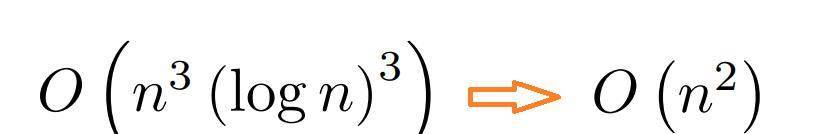## TinyGL（計算機圖形學）

1998 年，Bellard 在開發一個虛擬現實引擎項目的時候，需要用到OpenGL，這是一個用于渲染2D、3D矢量圖形的跨語言、跨平台的API，OpenGL的開源實現是Mesa，Bellard覺得Mesa太慢了，于是自己寫了一個，這就是TinyGL。

TinyGL實現了OpenGL的子集，比Mesa或者其他商用實現（如Solaris OpenWin OpenGL）快得多，占用的資源少得多，并且比任何一個都小幾個數量級，Bellard 再次展示了他在編寫高效 C 代碼方面的超高技能。

## FFmpeg（音頻視訊多媒體）

2000年，他化名Gérard Lantau，開始了他最重要和最受廣泛認可的項目之⼀：FFmpeg。## C語言混淆競賽

int m = 754974721， N， t[1 < 22]，="" a，="" *p，="" i，="" e="1">< 22，="" j，="" s，="" b，="" c，="" u；f="" （d）{="" for="" （s="1">< 23；="" s；="" s="" 2，="" d="d" *="" 1ll="" *="" d="" %="" m）="" if="" （s="">< n）="" for="" （p="t；" p="">< t="" +="" n；="" p="" +="s）" for="" （i="s，" c="1；" i；="" i--）="" b="*p" +="" p[s]，="" p[s]="（m" +="" *p="" -="" p[s]）="" *="" 1ll="" *="" c="" %="" m，="" *p++="b" %="" m，="" c="c" *="" 1ll="" *="" d="" %="" m；="" for="" （j="0；" i="">< n="" -="" 1；）="" {="" for="" （s="N" 2；="" ！（（j="" ^="s）" &="" s）；="" s="" 2）；="" if="" （++i="">< j）="" a="t[i]，" t[i]="t[j]，" t[j]="a；" }}main="" （）{="" *t="2；" u="N" =="" 1；="" while="" （e="" 2）="" {="" n="" *="2；" u="U" *="" 1ll="" *="" （m="" +="" 1）="" 2="" %="" m；="" f="" （362）；="" for="" （p="t；" p="">< t="" +="" n；）="" *p++="（*p" *="" 1ll="" **="" p="" %="" m）="" *="" u="" %="" m；="" f="" （415027540）；="" for="" （a="0，" p="t；" p="">< t="" +="" n；）="" a="" +="（6972593" &="" e="" ？="" 2="" ：="" 1）="" **="" p，="" *p++="a" %="" 10，="" a="" 10；="" }="" while="" （！*--p）；="" t--；="" while="" （p="">= t） printf （「%d」， *p--）；}

## TinyCC（最快的編譯器）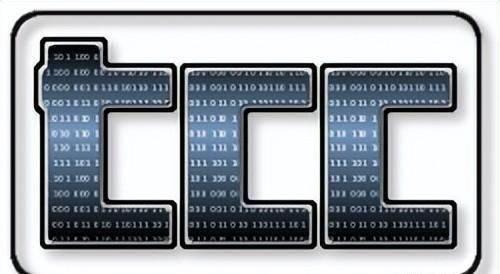## QEMU（模擬器）

2005年，Bellard又發布了一個爆炸性項目QEMU，這是一個開源的模擬器，可以用軟件的方式來模擬CPU，內存，I/O設備，給操作系統營造一個運行在硬件中的假象。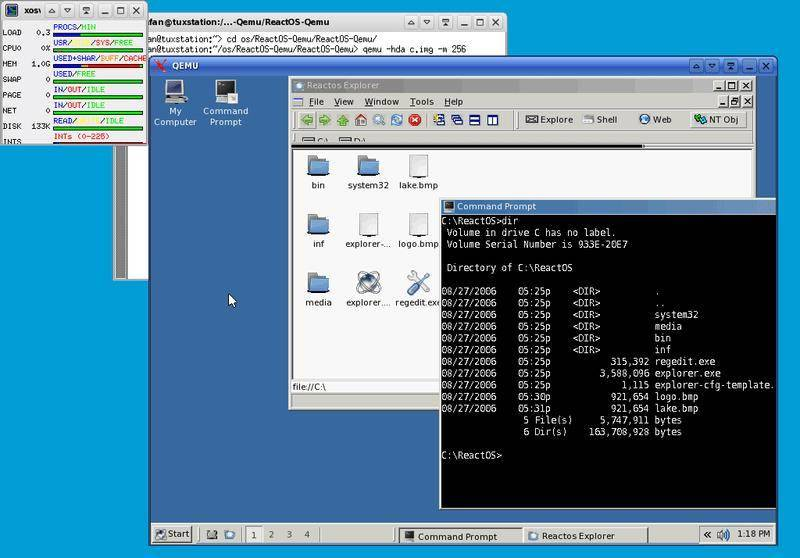## 創造PI的世界紀錄

2009年，Bellard又去玩數學了，他宣布把圓周率小數點后2.7萬億位以后，僅僅使用了一台普通的PC，創造了世界紀錄。

## JSLinux（模擬器）

2011年，他的興趣又轉到了JavaScript身上，居然用JavaScript寫了一個PC模擬器，讓Linux，Windows可以在瀏覽器中運行起來。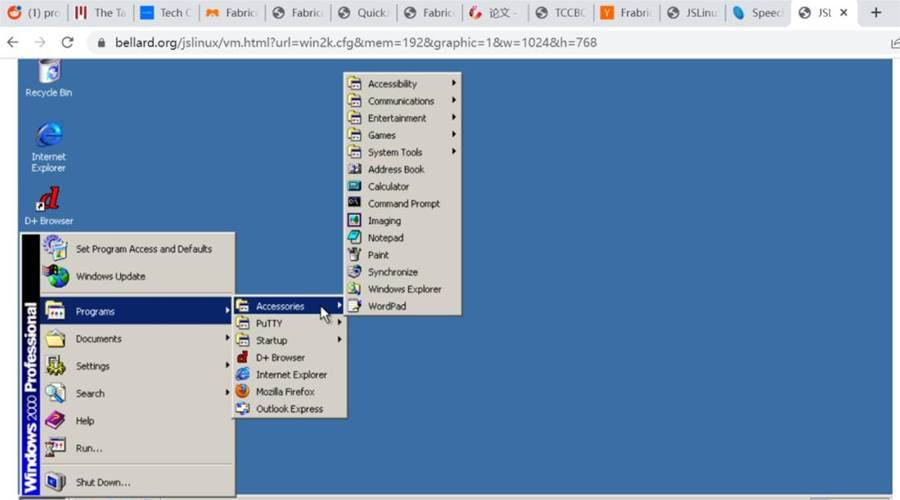## LTE軟基站（通信）

2012年，Bellard的興趣再次轉移，一個人花了10個月時間，在一台PC上居然實現了一個運行效率極高LTE軟基站。支持LTE TDD/FDD，NB-IoT、eMTC，最大可支持5載波2x2 MIMO或3載波4x4 MIMO。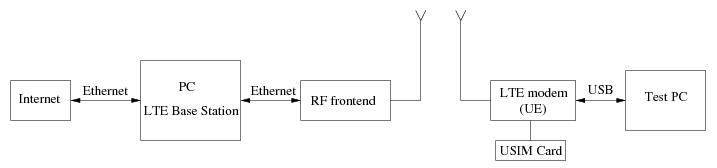## QuickJS （JavaScript解釋器）

2019年，Bellard發布了一個嵌入式的JavaScript執行引擎QuickJS。

QuickJS支持ES2020，小巧并且易于嵌入，只有幾個C文件，沒有任何其他外部依賴。

Bellard給人的印象是，他可以輕易進入一個他覺得有趣的領域，成為這個領域的專家，留下一個讓其他人愿意花費數年時間維護的軟件，自己則輕飄飄地離開，進入下一個領域。

Just for fun，這是Linus的口號，看來各個大神的追求都是一樣的啊。

Bellard對金錢或者名聲不感興趣（用化名做開源項目就是證明），他極少接受媒體的采訪，互聯網上他的資料非常少，遠不如Linus那樣聲名遠揚。

Bellard一定是穿越回來的，或者一定是在上帝模式下編程。